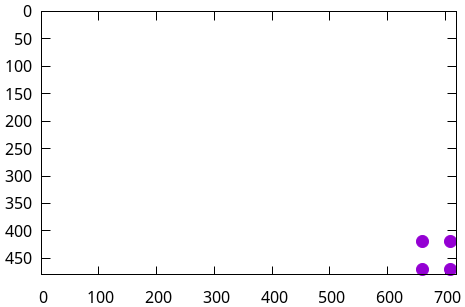# Overlay a transparent logo on a given video file using FFMPEG

Following FFMPEG command will accoumplish the same. Lets analyse the command.

ffmpeg -i video.mp4 -i img.jpg -filter_complex "scale=50:50,format=argb,geq=r='r(X,Y)':a='0.3*alpha(X,Y)'[tranimg];[tranimg]overlay=W-w-10:H-h-10" output.mp4

• -i - input files. Here there are two inputs, one video and one image.
• -filter_complex - It is for adding complex filters. In this case

scale=50:50,format=argb,geq=r='r(X,Y)':a='0.3*alpha(X,Y)'[tranimg];[tranimg]overlay=W-w-10:H-h-10

• Let check the applied filter.

scale=50:50,format=argb,geq=r='r(X,Y)':a='0.3*alpha(X,Y)'[tranimg]

In this case 1 means the second input, ie the image(First input is 0). All the following filters are applied on it.

• scale=50:50 - It scales the image to 50x50

• format=argb - changes the format to RGB

• geq=r='r(X,Y)':a='0.3*alpha(X,Y)' - It is for changing the opacity of the image. All geq requires an RGB or luminance expression to let the filter know that it is RGB or YUV. In this case setting red component to itself. Then changing the opacity of each pixel to 0.3 of itself.

• tranimg - Its just a name given to output

Now the overlay function overlays the output tranimg on video file at the given x and y co-ordinate.

[tranimg]overlay=W-w-10:H-h-10

The image is already scaled to 50x50. Now lets check the size of the video frame.

ffmpeg -i video.mp4 2>&1 | egrep -o "[0-9]+x[0-9]+ "
720x480


Video is 720x480, so the image will be overlayed at 660,420

• W-w-10 ie 720-50-10=660
• H-h-10 ie 480-50-10=420

considering image size is 50x50. All 4 points of image will be at co-ordinates :

X    Y
660 420
660 470
710 420
710 470


If we plot that, we can see the image comes at lower right corner of the video.For the transparency colorchannelmixer option also works. So you can replace :

geq=r='r(X,Y)':a='0.3*alpha(X,Y)'

with

colorchannelmixer=aa=0.3

ffmpeg -i video.mp4 -i img.jpg -filter_complex "scale=50:50,format=rgba,colorchannelmixer=aa=0.2[tranimg];[tranimg]overlay=W-w-10:H-h-10" -c:v libx265 -c:a aac output.mp4